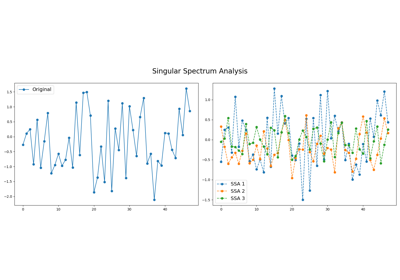# `pyts.decomposition`.SingularSpectrumAnalysis¶

class `pyts.decomposition.``SingularSpectrumAnalysis`(window_size=4, groups=None)[source]

Singular Spectrum Analysis.

Parameters: window_size : int or float (default = 4) Size of the sliding window (i.e. the size of each word). If float, it represents the percentage of the size of each time series and must be between 0 and 1. The window size will be computed as `max(2, ceil(window_size * n_timestamps))`. groups : None, int or array-like (default = None) The way the elementary matrices are grouped. If None, no grouping is performed. If an integer, it represents the number of groups and the bounds of the groups are computed as `np.linspace(0, window_size, groups + 1).astype('int64')`. If array-like, each element must be array-like and contain the indices for each group.

References

 [Rf17d82cd0544-1] N. Golyandina, and A. Zhigljavsky, “Singular Spectrum Analysis for Time Series”. Springer-Verlag Berlin Heidelberg (2013).

Examples

```>>> from pyts.datasets import load_gunpoint
>>> from pyts.decomposition import SingularSpectrumAnalysis
>>> X, _, _, _ = load_gunpoint(return_X_y=True)
>>> transformer = SingularSpectrumAnalysis(window_size=5)
>>> X_new = transformer.transform(X)
>>> X_new.shape
(50, 5, 150)
```

Methods

 `__init__`(self[, window_size, groups]) Initialize self. `fit`(self[, X, y]) Pass. `fit_transform`(self, X[, y]) Fit to data, then transform it. `get_params`(self[, deep]) Get parameters for this estimator. `set_params`(self, \*\*params) Set the parameters of this estimator. `transform`(self, X) Transform the provided data.
`__init__`(self, window_size=4, groups=None)[source]

Initialize self. See help(type(self)) for accurate signature.

`fit`(self, X=None, y=None)[source]

Pass.

Parameters: X ignored y Ignored self : object
`fit_transform`(self, X, y=None, **fit_params)

Fit to data, then transform it.

Fits transformer to X and y with optional parameters fit_params and returns a transformed version of X.

Parameters: X : numpy array of shape [n_samples, n_features] Training set. y : numpy array of shape [n_samples] Target values. **fit_params : dict Additional fit parameters. X_new : numpy array of shape [n_samples, n_features_new] Transformed array.
`get_params`(self, deep=True)

Get parameters for this estimator.

Parameters: deep : bool, default=True If True, will return the parameters for this estimator and contained subobjects that are estimators. params : mapping of string to any Parameter names mapped to their values.
`set_params`(self, **params)

Set the parameters of this estimator.

The method works on simple estimators as well as on nested objects (such as pipelines). The latter have parameters of the form `<component>__<parameter>` so that it’s possible to update each component of a nested object.

Parameters: **params : dict Estimator parameters. self : object Estimator instance.
`transform`(self, X)[source]

Transform the provided data.

Parameters: X : array-like, shape = (n_samples, n_timestamps) X_new : array-like, shape = (n_samples, n_splits, n_timestamps) Transformed data. `n_splits` value depends on the value of `groups`. If `groups=None`, `n_splits` is equal to `window_size`. If `groups` is an integer, `n_splits` is equal to `groups`. If `groups` is array-like, `n_splits` is equal to the length of `groups`. If `n_split=1`, `X_new` is squeezed and its shape is (n_samples, n_timestamps).

## Examples using `pyts.decomposition.SingularSpectrumAnalysis`¶Singular Spectrum Analysis